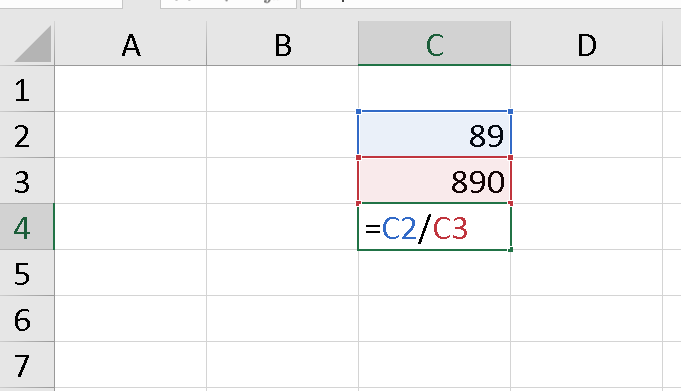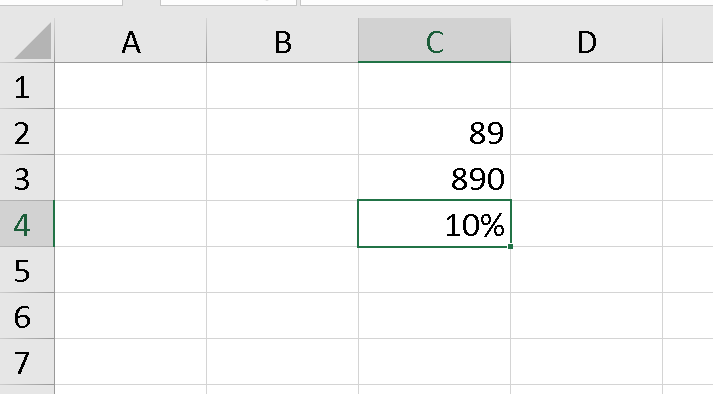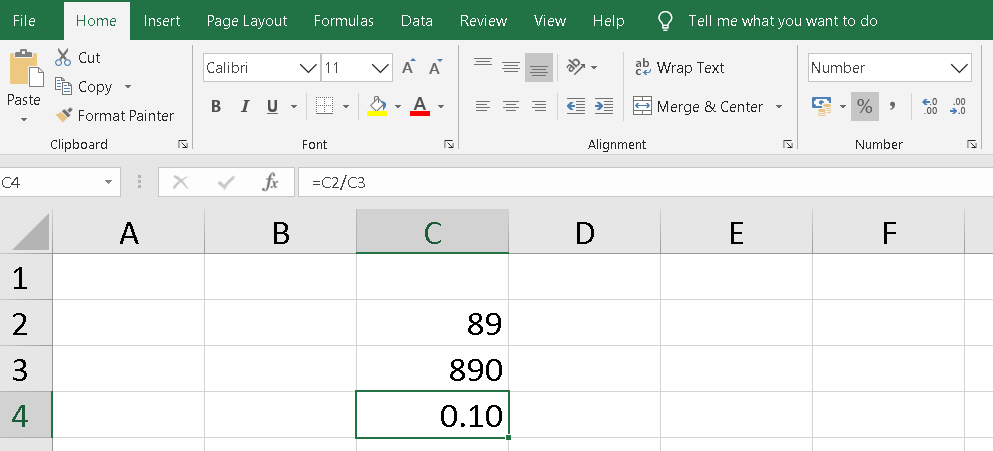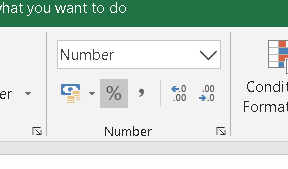# How to Find the Percentage of Two Cells in Microsoft Excel?

• Difficulty Level : Basic
• Last Updated : 18 Jul, 2021

If you want to find the percentage of two cells in Microsoft Excel, Simply select an empty cell, where you want to display the percentage of the two cells.

Type in the following formula in the selected cell :

`=address of cell1/address of cell2`

For example, I want to print out the percentage of C2 and C3, so the formulae will be: =C2/C3, in an empty cell C4.As soon as you click on the return key, You will get your percentage calculated in cell C4.However, if a decimal value is displayed in the cell. Like 0.10, Simply press the shortcut key Ctrl+Shift+% to format as a percent or convert the numeric value into the percentage style and press the Return key.

The Decimal value will be converted to a percentage.BeforeAfter

You can also go to the Number section under the Home tab and press the % Symbol to convert to percentage format.% symbol under the Number listbox

My Personal Notes arrow_drop_up